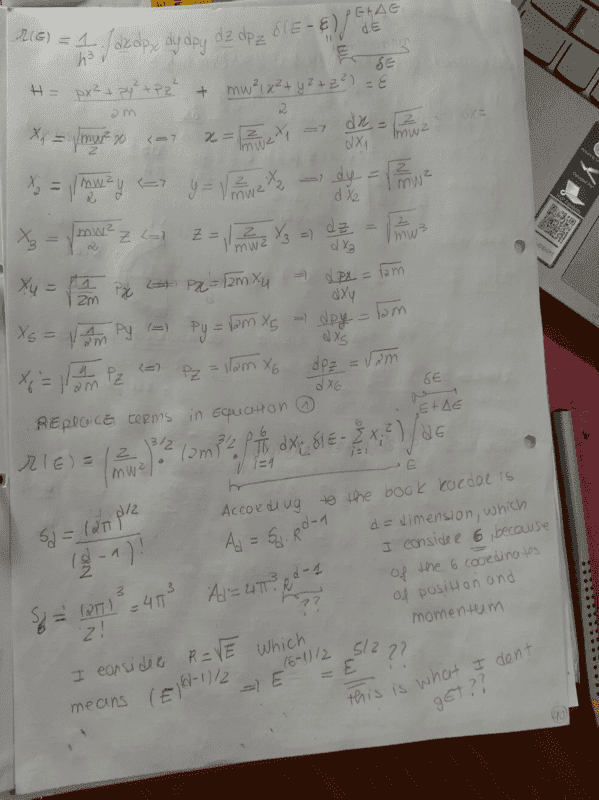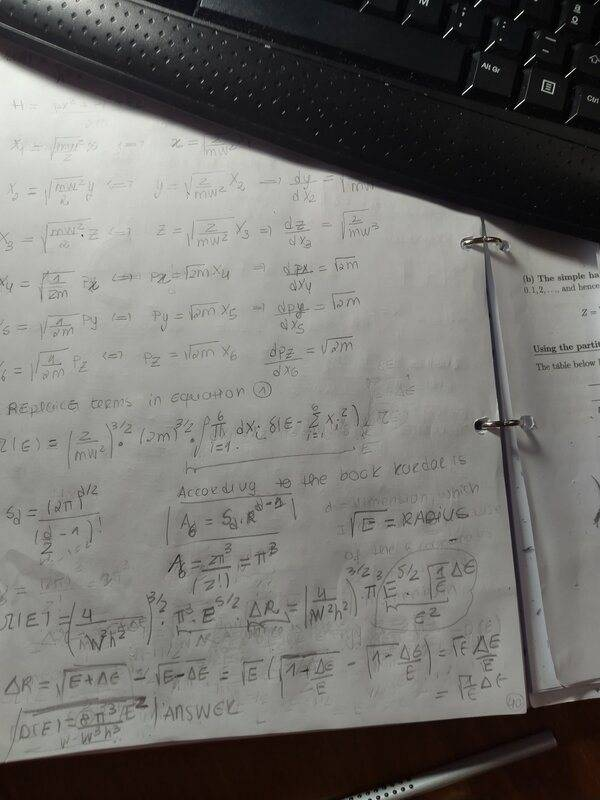# Density of states of one three-dimensional classical harmonic oscillator

• anaisabel
In summary, there was a conversation about hypersphere dimension confusion and the dimensions of a "regular" sphere in 3D space. The surface of a sphere is 2-dimensional, making a sphere in 3D space a 2-sphere. The hypersphere in a 6D space is a 5-sphere. There was a discussion about formulas and using the appropriate dimensions, with an emphasis on the inverse square root factor. It was mentioned that this factor may have been forgotten, leading to some errors in the calculations. A textbook was referenced, where the integral for the volume of a hypersphere is considered to be in 3D dimensions. However, in the final formula, only 3D is used. f

#### anaisabel

Homework Statement
I am trying to determine the density of states using phase space volume. In my solution I have managed to determine the constants and the variation of energy dE, so the term I cant figure out is the integral of the coordinates. According to my lectures and the example I did of N classical harmonic oscillators (2 dimensional), I can determine the integral as the area of 3 dimensional hypersphere, however when I apply the formula I have E^(5/2) and the exercise I am trying to solve says that the final result is D(E)=aE^(2), a is a constant. I dont know what I am doing wrong.
Relevant Equations
D(E) = dΩ/dEia

Lol when I saw this I thought "that's going to be hypersphere dimension confusion right?" and indeed it was. I've been there, I feel for you, it caused me a lot of headache.

This is a very common error. What are the dimensions of a "regular" sphere in 3d space? It's 3 right? Wrong! The surface is what is called a sphere. The surface is 2 dimensional. So a sphere in 3d space is a 2-sphere. The circle is a 1-sphere. The hypersphere in your 6d space is a 5-sphere, not a 6 sphere. Notation is annoying sometimes!

Well, I understand that but coming to that conclusion has to come from the calculations right? I know I am integrating in a 6 dimensional space, dxdydzdpxdpydpz, so when applying the formula I have to use 6d not 5d,because of the integral. But later on after thinking a bit more I realized that I didnt take in account the variation of the radius, which is sqrt(1/E)*ΔE. If I multiply that by (E)^5/2, I obtain the E^2. I think I can do it, like this but I am not sure. And but the constants arent right either, because after finishing the calculations I am off by a factor of 1/2.

so when applying the formula I have to use 6d not 5d,because of the integral
Which formula are you referring to? There is a formula which gives you the area of an n-sphere. In this case, you have a 5-sphere, not a 6-sphere, because you are dealing with a 6 dimensional phase space, and in particular with the surface of constant energy, which is a surface of constant radius, and the surface of constant radius in n dimensions has n-1 dimensions. dΩ will be the surface of the 5-sphere times the variation of radius, right? Writing it as a variation of E does indeed involve a factor of 1/sqrtE. The surface of the sphere is proportional to R^5, so E^(5/2). Multiply it with 1/sqrtE, and you get E^2 as you would expect. I think you may have mixed up your formulas somewhere.

If you look at textbooks , when writing the integral they always use the dimension that is applied to the situation. So in the case of the ideal gas is 3d and in the final formula they use 3d not 2d, I will show what I did, it is the bottom of the page. If i use 5d instead of 6d, I will not obtain pi^3 and i have to, according to the solutions. However in my asnwer I have 8 and in the solutions they have 4.If you look at textbooks , when writing the integral they always use the dimension that is applied to the situation
Can you show me the relevant part in a textbook? You may have mixed up an index somewhere, some books define the formulas kinda weird and it's easy to get your wires crossed. Regardless, you are dealing with a 5-sphere, since the space is 6 dimensional. A 5-sphere's surface is proportional to R^5, just like the surface of a 1-sphere (perimeter of a circle) is proportional to R.

Oooooh now I figured out where you went wrong. It really is just the fact that you forgot the 1/sqrtE factor, but you also mixed up some other stuff. The solid angle formula corresponds to d=6, but the solid angle for d gives you the area of a (d-1)-sphere so that's why there was miscommunication. So turns out that it really is just that you forgot the inverse square root factor. I thought you hadn't forgotten it at first because I saw the (d-1)/2 and I thought that was where the -1/2 was coming from.

Additionally, you said that even if you do that, you are still off by a factor of 1/2. This may be because dR=(1/2)*(1/sqrtE)dE and you forgot the 1/2, so check that.

Can you show me the relevant part in a textbook? You may have mixed up an index somewhere, some books define the formulas kinda weird and it's easy to get your wires crossed. Regardless, you are dealing with a 5-sphere, since the space is 6 dimensional. A 5-sphere's surface is proportional to R^5, just like the surface of a 1-sphere (perimeter of a circle) is proportional to R.
the book in question is kardar particles, section 4.4, ideal gas. Kardar considers the integral the volume of hypersphere of dimension d with thickness ΔR. In that case, the general formula for N particles, is 3D, because the integral doesn't depend on the position , so your only integrating the momentum, which is 3D dimensions, so in the final formula you 3D. In the calculations kardar never considers 2D, he comes to that conclusion in the end.

Additionally, you said that even if you do that, you are still off by a factor of 1/2. This may be because dR=(1/2)*(1/sqrtE)dE and you forgot the 1/2, so check that.
I think my mistake is considering a range of E+ ΔE and E-ΔE, which in that case. it doesn't appear the (1/2), but if I consider the range E+ ΔE and E , then that factor (1/2) appears, so I think that's the issue.

the book in question is kardar particles, section 4.4, ideal gas. Kardar considers the integral the volume of hypersphere of dimension d with thickness ΔR. In that case, the general formula for N particles, is 3D, because the integral doesn't depend on the position , so your only integrating the momentum, which is 3D dimensions, so in the final formula you 3D. In the calculations kardar never considers 2D, he comes to that conclusion in the end.
Oh, I have that book, I am looking at it and yeah, he does notate it the same way you do. It's not a "conclusion", he just calls a (d-1)-sphere a "d dimensional sphere". It's all kina weird and can be confusing sometimes but yeah, your formulas are fine, you just forgot to take the correct variation in terms of E into account.

I think my mistake is considering a range of E+ ΔE and E-ΔE, which in that case. it doesn't appear the (1/2), but if I consider the range E+ ΔE and E , then that factor (1/2) appears, so I think that's the issue.
Very likely, I can't check it right now.

Oh, I have that book, I am looking at it and yeah, he does notate it the same way you do. It's not a "conclusion", he just calls a (d-1)-sphere a "d dimensional sphere". It's all kina weird and can be confusing sometimes but yeah, your formulas are fine, you just forgot to take the correct variation in terms of E into account.

Very likely, I can't check it right now.
no problem, thanks for helping:)

•AndreasC